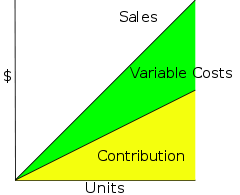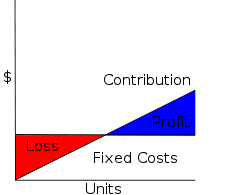# Contribution margin

﻿
Contribution marginDecomposing Sales as Contribution plus Variable Costs. In the *****Cost-Volume-Profit Analysis model, costs are linear in volume.

In cost-volume-profit analysis, a form of management accounting, contribution margin is the marginal profit per unit sale. It is a useful quantity in carrying out various calculations, and can be used as a measure of operating leverage. Typically, high contribution margins are prevalent in the labour-intensive tertiary sector while low contribution margins are prevalent in the capital-intensive industrial sector.

## Definition

The Unit Contribution Margin (C) is Unit Revenue (Price, P) minus Unit Variable Cost (V):

C = P − V

The Contribution Margin Ratio is the percentage of Contribution over Total Revenue, which can be calculated from the unit contribution over unit price or total contribution over Total Revenue:$\frac{\text{C}}{\text{P}}=\frac{\text{P}-\text{V}}{\text{P}}=\frac{\text{Unit Contribution Margin}}{\text{Price}}=\frac{\text{Total Contribution Margin}}{\text{Total Revenue}}$

For example, if the price is Rs10 and the unit variable cost is Rs2, then the unit contribution margin is Rs8, and the contribution margin ratio is Rs8/Rs10 = 80%.

## ExplanationProfit and Loss as Contribution minus Fixed Costs.

Contribution margin can be thought of as the fraction of sales that contributes to the offset of fixed costs. Alternatively, unit contribution margin is the amount each unit sale adds to profit: it's the slope of the Profit line.

Cost-Volume-Profit Analysis (CVP): assuming the linear CVP model, the computation of Profit and Loss (Net Income) reduces as follows:\begin{align} \text{PL} &= \text{TR} - \text{TC}\\ &= \left(\text{C}+\text{V}\right)\times \text{X} - \left(\text{TFC} + \text{V} \times \text{X}\right)\\ &= \text{C} \times \text{X} - \text{TFC} \end{align}

where TC = TFC + TVC is Total Cost = Total Fixed Cost + Total Variable Cost and X is Number of Units. Thus Profit is Unit Contribution times Number of Units, minus the Total Fixed Costs.

The above formula is derived as follows:

From the perspective of the matching principle, one breaks down the revenue from a given sale into a part to cover the Unit Variable Cost, and a part to offset against the Total Fixed Costs. Breaking down Total Costs as:$\text{TC} = \text{TFC} + \text{V} \times \text{X}$

one breaks down Total Revenue as:\begin{align} \text{TR} &= \text{P} \times \text{X}\\ &= \bigl(\left(\text{P} - \text{V} \right)+\text{V}\bigr)\times \text{X}\\ &= \left(\text{C}+\text{V}\right)\times \text{X}\\ &= \text{C}\times\text{X} + \text{V}\times \text{X} \end{align}

Thus the Total Variable Costs$\text{TVC} = \text{V} \times \text{X}$ offset, and the Net Income (Profit and Loss) is Total Contribution Margin minus Total Fixed Costs:\begin{align} \text{PL} &= \text{TR} - \text{TC}\\ &= \left(\text{C}+\text{V}\right)\times \text{X} - \left(\text{TFC} + \text{V} \times \text{X}\right)\\ &= \text{C} \times \text{X} - \text{TFC}\\ &= \text{TCM} - \text{TFC} \end{align}

## Applications

Contribution arises in Cost-Volume-Profit Analysis, where it simplifies calculation of Net Income, and especially break even analysis.

Given the contribution margin, a manager can easily compute breakeven and target income sales, and make better decisions about whether to add or subtract a product line, about how to price a product or service, and about how to structure sales commissions or bonuses.

Contribution margin analysis is a measure of operating leverage: it measures how growth in sales translates to growth in profits.

The contribution margin is computed by using a contribution income statement: a management accounting version of the income statement that has been reformatted to group together a business's fixed and variable costs.

Contribution is different than Gross Margin in that a contribution calculation seeks to separate out variable costs (included in the contribution calculation) from fixed costs (not included in the contribution calculation) on the basis of economic analysis of the nature of the expense whereas gross margin is determined using accounting standards. Calculating the contribution margin is an excellent tool for managers to help determine whether to keep or drop certain aspects of the business. For example, a production line with positive contribution margin should be kept even it causes negative total profit, when the contribution margin offsets part of the fixed cost. However, it should be dropped if contribution margin is negative because the company would suffer from every unit it produces.

The contribution margin analysis is also applicable when the tax authority performs tax investigation, by identifying target interviewee who has unusual high contribution margin ratio then other companies in the same industry.

Contribution margin is also one of the factors to judge whether a company has monopoly power in competition law, such as use of the Lerner Index test.

## Examples

Here's an example of a contribution format income statement:

 Sales $462,452 Less Variable Costs: Cost of Goods Sold Sales Commissions Delivery Charges$ 230,934 $58,852$ 13,984 Total Variable Costs $303,770 Contribution Margin (34%)$ 158,682 Less Fixed Costs: Advertising Depreciation Insurance Payroll Taxes Rent Utilities Wages $1,850$ 13,250 $5,400$ 8,200 $9,600$ 17,801 $40,000 Total Fixed Costs$ 96,101 Net Operating Income $62,581 The Beta Company's contribution margin for the year was 34 percent. This means that, for every dollar of sales, after the costs that were directly related to the sales were subtracted, 34 cents remained to contribute toward paying for the indirect costs and for profit. Contribution format income statements can be drawn up with data from more than one year's income statements, when a person is interested in tracking contribution margins over time. Perhaps even more usefully, they can be drawn up for each product line or service. Here's an example, showing a breakdown of Beta's three main product lines: Line A Line B Line C Sales$120,400 $202,050$140,002
Less Variable Costs:
Cost of Goods Sold $70,030$100,900 $60,004 Sales Commissions$18,802 $40,050$0
Delivery Charges $900$ 8,084 $5,000 Total Variable Costs$ 89,732 $149,034$ 65,004
Contribution Margin $30,668$ 53,016 $74,998 percentage 25% 26% 54% Although this shows only the top half of the contribution format income statement, it's immediately apparent that Product Line C is Beta's most profitable one, even though Beta gets more sales revenue from Line B(which is also an example of what is called Partial Contribution Margin - an income statement that references only variable costs). It appears that Beta would do well by emphasizing Line C in its product mix. Moreover, the statement indicates that perhaps prices for line A and line B products are too low. This is information that can't be gleaned from the regular income statements that an accountant routinely draws up each period. ### Contribution Margin as a measure of efficiency in the operating room The following discussion focuses on Contribution Margin (mean) per operating room hour in the operating room and how it relates to operating room efficiency. FIGURE: Metric Measure for OR efficiencyMacario A. Are Your Hospital Operating Rooms "Efficient"? Anesthesiology 2006; 105:237-40. Metric Measures 0 1 2 Excess Staffing Costs >10% 5-10% <5% Start-time tardiness (mean tardiness for elective cases/day) >60 min 45-60 min <45 min Case cancellation rate >10% 5-10% <5% Post Anesthesia Care Unit (PACU) admission delays (% workdays with at least one delay in PACU admission) >20% 10-20% <10% Contribution Margin (mean) per operating room hour <$1,000/hr $1–2,000/hr >$2,000/hr
Operating Room Turnover Time (mean setup and cleanup turnover times for all cases) >40 min 25-40 min <25 min
Prediction Bias (bias in case duration estimates per 8 hours of operating room time) >15 min 5-15 min <5 min
Prolonged turnovers (%turnovers > 60 min) >25% 10-25% <10%

A surgical suite can schedule itself efficiently but fail to have a positive contribtution margin if many surgeons are slow, use too many instruments or expensive implants, etc. These are all measured by the contribution margin per OR hr. The contribution margin per hour of OR time is the hospital revenue generated by a surgical case, less all the hospitalization variable labor and supply costs. Variable costs, such as implants, vary directly with the volume of cases performed.

This is because fee-for-service hospitals have a positive contribution margin for almost all elective cases mostly due to a large percentage of OR costs being fixed. For USA hospitals not on a fixed annual budget, contribution margin per OR hour averages one to two thousand USD per OR hour.

Wikimedia Foundation. 2010.

### Look at other dictionaries:

• Contribution Margin — A cost accounting concept that allows a company to determine the profitability of individual products. It is calculated as follows: Product Revenue Product Variable Costs Product Revenue The phrase contribution margin can also refer to a per unit …   Investment dictionary

• contribution margin — contribution …   Accounting dictionary

• Contribution margin — The difference between variable revenue and variable cost. The New York Times Financial Glossary …   Financial and business terms

• contribution margin — The difference between variable revenue and variable cost. Bloomberg Financial Dictionary …   Financial and business terms

• Contribution Margin — ⇡ Deckungsbeitrag …   Lexikon der Economics

• contribution margin — /ˌkɒntrɪ bju:ʃ(ə)n ˌmɑ:dʒɪn/ noun the difference between the revenue generated by a product and its total variable costs …   Marketing dictionary in english

• Contribution margin-based pricing — maximizes the profit derived from an individual product, based on the difference between the product s price and variable costs (the product s contribution margin per unit), and on one’s assumptions regarding the relationship between the… …   Wikipedia

• contribution margin ratio — contribution to sales ratio; = production–volume ratio; = profit–volume ratio The ratio of the contribution per product to the sales value, often expressed as a percentage. It is a way of ranking a range of products in terms of their relative… …   Accounting dictionary

• Маржинальная прибыль (CONTRIBUTION MARGIN, MARGINAL CONTRIBUTION)  — Разность между доходом от реализации и переменными затратами. Для достижения точки безубыточности маржинальная прибыль должна покрыть постоянные затраты. См. также Доля маржинальной прибыли (Contribution Margin Ratio); Маржинальная прибыль на… …   Словарь терминов по управленческому учету

• Маржинальная прибыль на единицу реализованной продукции (CONTRIBUTION MARGIN PER UNIT)  — Маржинальная прибыль, рассчитанная на единицу продукции. См. Маржинальная прибыль (Contribution Margin) …   Словарь терминов по управленческому учету